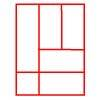#### You may also like### Bracelets

Investigate the different shaped bracelets you could make from 18 different spherical beads. How do they compare if you use 24 beads?### Cut and Make

Cut a square of paper into three pieces as shown. Now,can you use the 3 pieces to make a large triangle, a parallelogram and the square again?### Is a Square a Rectangle?

How many rectangles can you find in this shape? Which ones are differently sized and which are 'similar'?

# Numerically Equal

## Numerically Equal

I want to draw a square in which the perimeter is numerically equal to the area.Of course, the perimeter will be measured in units of length, for example, centimetres (cm) while the area will be measured in square units, for example, square centimetres (cm$^2$).

What size square will I need to draw?

What about drawing a rectangle that is twice as long as it is wide which still has a perimeter numerically equal to its area?

Can They Be Equal? offers a suitable extension to this problem.

### Why do this problem?

This problem requires learners to consider area and perimeter at the same time and to know the difference between the two. It provides a good opportunity to talk about different ways of reaching a solution.

### Possible approach

When introducing this problem it is necessary to emphasise that although the perimeter and the area will be 'numerically equal', that is, the same number, they are not the same measurement because the perimeter will be measured in units of length, such as centimetres (cm), while the area will be measured in square units, such as square centimetres (cm$^2$).

Once children have understood the task, they could work in pairs so that they are able to talk through their ideas with a partner. Having squared paper available would be a good idea, although not all learners will choose to use it.

It would be interesting and instructive to listen to the way that the various pairs are working on the problem because there are many possible approaches and in the plenary, you can draw this out. Give pairs time to explain how they reached an answer - for example, they might have drawn squares and calculated area/perimeter until they found one that works; they might have taken a purely numerical approach by looking at square numbers and dividing them by four. In either case, some pupils might notice patterns in the numbers obtained which helped them to know they were getting close. You could ask the class to comment about the advantages of each different way and you could then split them into small groups to produce posters about each method for display.

### Key questions

What is the perimeter of this square? What is its area?
How will you know you haven't missed out the square that works?

### Possible extension

The second half of the problem could be used as an extension and could be continued with a question such as: "What about a rectangle that is three times as long as it is wide?".

### Possible support

Being able to draw squares on squared paper, and record the area and perimeter of each one, should help all pupils access this problem.